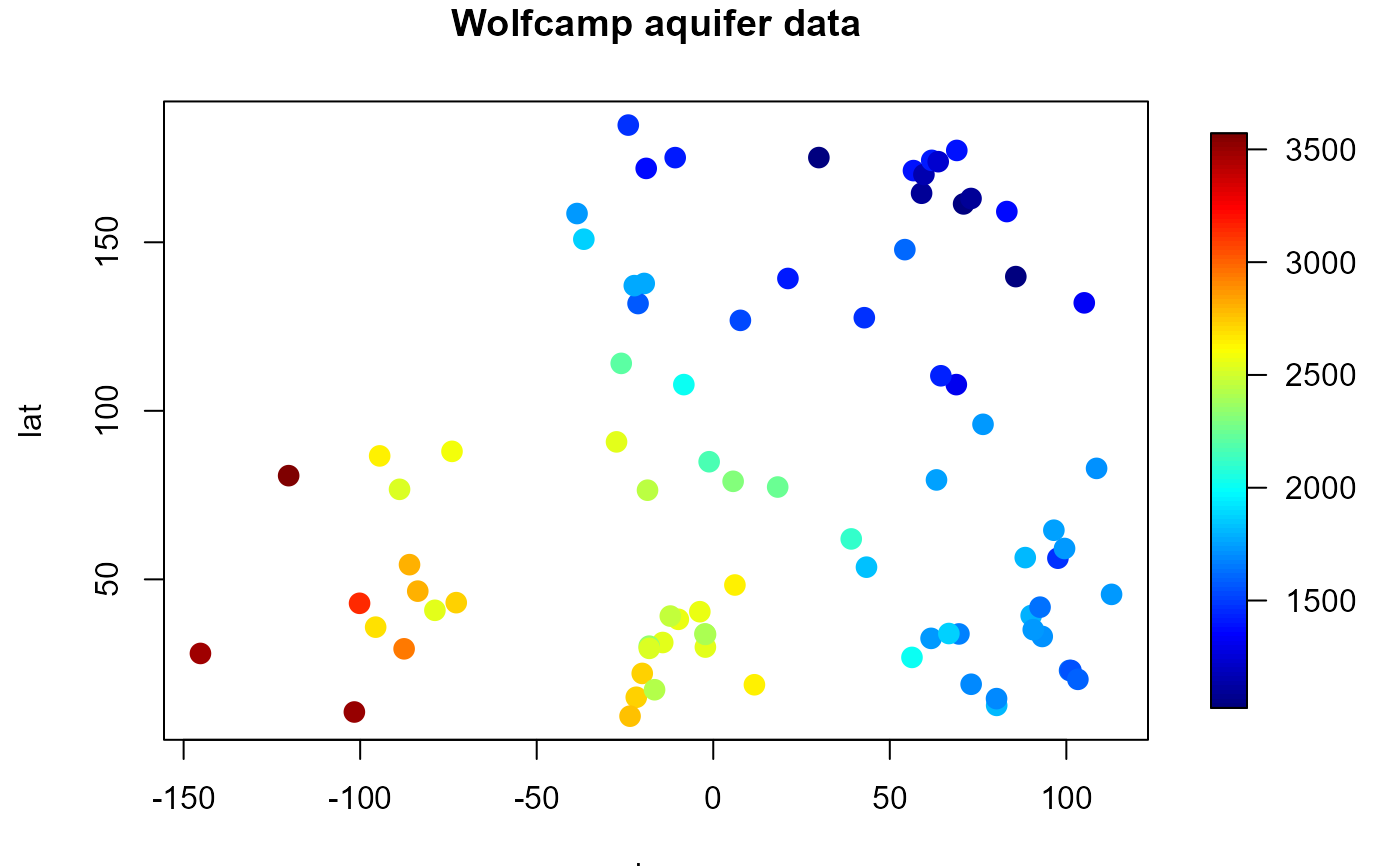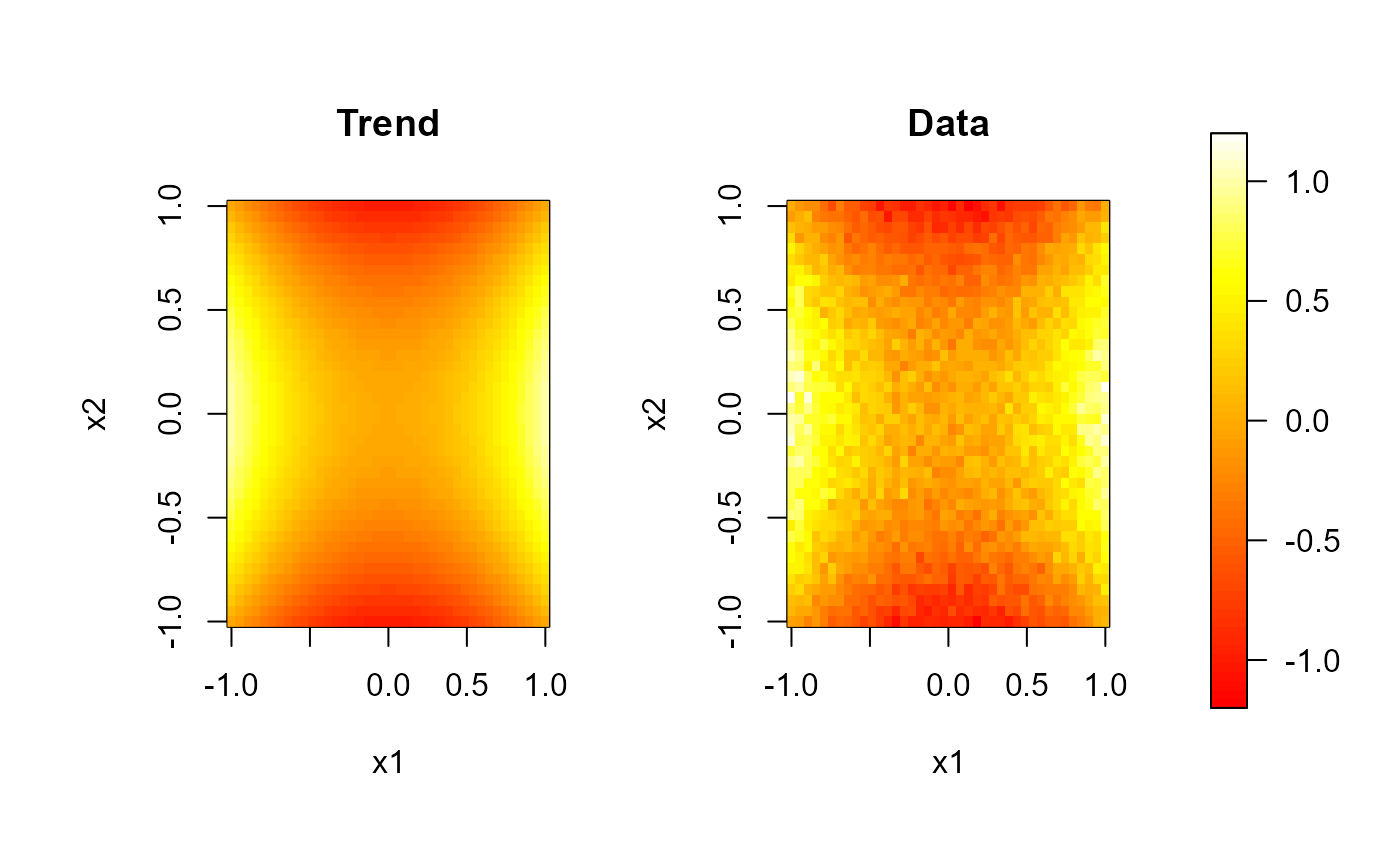splot is designed to combine a standard R plot with a legend representing a (continuous) color scale. This is done by splitting the plotting region into two parts. Keeping one for the main chart and putting the legend in the other.

sxxxx functions (spoints, simage and spersp) draw the corresponding high-level plot (xxxx) with a legend strip for the color scale.

These functions are based on function image.plot of package fields, see its documentation for additional information.

jet.colors and hot.colors create a color table useful for contiguous color scales and scolor assigns colors to a numerical vector.

splot(
slim = c(0, 1),
col = jet.colors(128),
breaks = NULL,
horizontal = FALSE,
legend.shrink = 0.9,
legend.width = 1.2,
legend.mar = ifelse(horizontal, 3.1, 5.1),
legend.lab = NULL,
bigplot = NULL,
smallplot = NULL,
lab.breaks = NULL,
axis.args = NULL,
legend.args = NULL,
)

scolor(s, col = jet.colors(128), slim = range(s, finite = TRUE))

jet.colors(n)

hot.colors(n, rev = TRUE)

## Arguments

slim

limits used to set up the color scale.

col

color table used to set up the color scale (see image for details).

breaks

(optional) numeric vector with the breakpoints for the color scale: must have one more breakpoint than col and be in increasing order.

horizontal

logical; if FALSE (default) legend will be a vertical strip on the right side. If TRUE the legend strip will be along the bottom.

legend.shrink

amount to shrink the size of legend relative to the full height or width of the plot.

legend.width

width in characters of the legend strip. Default is 1.2, a little bigger that the width of a character.

legend.mar

width in characters of legend margin that has the axis. Default is 5.1 for a vertical legend and 3.1 for a horizontal legend.

legend.lab

label for the axis of the color legend. Default is no label as this is usual evident from the plot title.

bigplot

plot coordinates for main plot. If not passed these will be determined within the function.

smallplot

plot coordinates for legend strip. If not passed these will be determined within the function.

lab.breaks

if breaks are supplied these are text string labels to put at each break value. This is intended to label axis on a transformed scale such as logs.

axis.args

additional arguments for the axis function used to create the legend axis (see image.plot for details).

legend.args

arguments for a complete specification of the legend label. This is in the form of list and is just passed to the mtext function. Usually this will not be needed (see image.plot for details).

logical; if TRUE the legend strip is just added to the existing plot.

s

values to be converted to the color scale.

n

number of colors (>= 1) to be in the palette.

rev

logical; if TRUE, the palette is reversed (decreasing overall luminosity).

## Value

splot invisibly returns a list with the following 3 components:

bigplot

plot coordinates of the main plot. These values may be useful for drawing a plot without the legend that is the same size as the plots with legends.

smallplot

plot coordinates of the secondary plot (legend strip).

old.par

previous graphical parameters (par(old.par) will reset plot parameters to the values before entering the function).

jet.colors and hot.colors return a character vector of colors (similar to heat.colors or terrain.colors; see rgb).

## Details

scolor converts a real valued vector to a color scale. The range slim is divided into length(col) + 1 pieces of equal length. Values which fall outside the range of the scale are coded as NA.

jet.colors generates a rainbow style color table similar to the MATLAB (TM) jet color scheme. It may be appropriate to distinguish between values above and below a central value (e.g. between positive and negative values).

hot.colors generates a color table similar to the MATLAB (TM) hot color scheme (reversed by default). It may be appropriate to represent values ranging from 0 to some maximum level (e.g. density estimation). The default value rev = TRUE may be adecuate to grayscale convertion.

## Side Effects

After exiting, the plotting region may be changed (par("plt")) to make it possible to add more features to the plot.

spoints, simage, spersp, image, image.plot.

## Author

Based on image.plot function from package fields: fields, Tools for spatial data. Copyright 2004-2013, Institute for Mathematics Applied Geosciences. University Corporation for Atmospheric Research.

Modified by Ruben Fernandez-Casal <rubenfcasal@gmail.com>.

## Examples

# Plot equivalent to spoints():
splot(slim = scale.range)
with( aquifer, plot(lon, lat, col = scolor(head, slim = scale.range),
pch = 16, cex = 1.5, main = "Wolfcamp aquifer data"))# Multiple plots with a common legend:
# regularly spaced 2D data...
set.seed(1)
nx <- c(40, 40) # ndata =  prod(nx)
x1 <- seq(-1, 1, length.out = nx)
x2 <- seq(-1, 1, length.out = nx)
trend <- outer(x1, x2, function(x,y) x^2 - y^2)
y <- trend + rnorm(prod(nx), 0, 0.1)
scale.range <- c(-1.2, 1.2)
scale.color <- heat.colors(64)
# 1x2 plot with some room for the legend...
old.par <- par(mfrow = c(1,2), omd = c(0.05, 0.85, 0.05, 0.95))
image( x1, x2, trend, zlim = scale.range, main = 'Trend', col = scale.color)
image( x1, x2, y, zlim = scale.range, main = 'Data', col = scale.color)
par(old.par)
# the legend can be added to any plot...
splot(slim = scale.range, col = scale.color, add = TRUE)## note that argument 'zlim' in 'image' corresponds with 'slim' in 'sxxxx' functions.